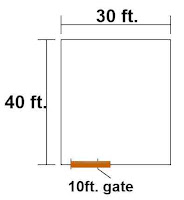### Estimating block masonry of compound wall. / Estimating & costing of a compound wall ( part 6 ).

Let us build this compound wall of 6" thickness having 6 ft. height as shown in the drawing.Plan of the compound wall.

The total length of the compound wall

= site perimeter - gate length

= (40ft. × 2 nos.) + (30 ft. × 2 nos.) - 10 ft.

= 80 ft. + 60 ft. -10 ft.

= 130 ft.

The total length of the block masonry

= compound wall length - (no. of columns × width of a single column.)

= 130 ft. - (14 nos.× 0.75 ft.)

= 130 ft. - 10.5 ft.

= 119.5 ft.

Height of the block masonry

= compound wall height - coping thickness

= 6 ft - 0.33 ft.

= 5.67 ft.

7. The volume of the block masonry

= length × height × thickness

= 119.5 ft. × 5.67 ft. × 0.5 ft.

= 338.7825 cu ft.

Number of concrete blocks required

= 210 nos. × ( 338.78 cu ft. ÷ 100 cu ft.)

= 712 nos.

The number of cement bags required

= 1.038 bags × ( 338.78 cu ft. ÷ 100 cu ft.)

=3.516 bags.

The volume of sand required

= 7.634 cu ft. × ( 338.78 cu ft. ÷ 100 cu ft.)

= 25.86 cu ft.

Note: The above-given quantity is taken from the article "Calculating the quantity of materials in a 100 cubic ft. block wall."

BACK 👈 PART 5          CONTINUED 👉 PART 7